#### 3D angular velocity

Now consider the rotation of a 3D rigid body. Recall from Section 3.3 that Euler's rotation theorem implies that every 3D rotation can be described as a rotation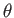about an axis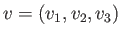though the origin. As the orientation of the body changes over a short period of time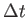, imagine the axis that corresponds to the change in rotation. In the case of the merry-go-round, the axis would be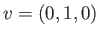. More generally,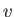could be any unit vector.

The 3D angular velocity is therefore expressed as a 3D vector: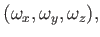(8.15)

which can be imagined as taking the original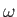from the 2D case and multiplying it by the vector. This weights the components according to the coordinate axes. Thus, the components could be considered as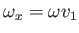,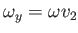, and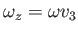. The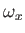,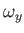, and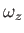components also correspond to the rotation rate in terms of pitch, roll, and yaw, respectively. We avoided these representations in Section 3.3 due to noncommutativity and kinematic singularities; however, it turns out that for velocities these problems do not exist . Thus, we can avoid quaternions at this stage.

Steven M LaValle 2020-11-11